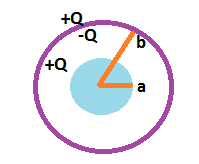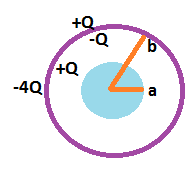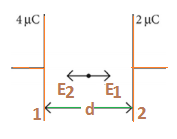# JEE Main Electrostatics Previous Year Questions with Solutions

Electrostatics is the branch of physics that deals with the study of the properties of stationary or slow-moving electric charges. According to Coulomb’s law, there is a force of attraction between these charges. Electrostatically induced forces seem to be rather weak.

Coulomb’s law: Coulomb’s law states that like charges repel and unlike charges attract, with a force equal to the product of the charges and inversely proportional to the square of the distance between them.

Electric field: Electric fields can be visualized using electric field lines. These field lines start from the positive charge and end at the negative charge. They are parallel to the direction of the field. The density of these field lines is a measure of the magnitude of the electric field at any given point.

## JEE Main Previous Year Solved Questions on Electrostatics

Q1: Three charges +Q, q, +Q are placed respectively, at distance 0, d/2 and d from the origin, on the x-axis. If the net force experienced by +Q placed at x = 0 is zero, then value of q is

(a) +Q/4

(b) –Q/2

(c) +Q/2

(d) –Q/4

Solution

QQ/d2 + Qq/(d/2)2 =0

Q + 4q = 0

or q = -Q/4

Q2: A parallel plate capacitor having capacitance 12 pF is charged by a battery to a potential difference of 10 V between its plates. The charging battery is now disconnected and a porcelain slab of dielectric constant 6.5 is slipped between the plates. The work done by the capacitor on the slab is

(a) 508 pJ

(b) 692 pJ

(c) 560 pJ

(d) 600 pJ

Solution

Work done = Change in energy stored in the system

W = (Q2/2C1) – (Q2/2C2)

Q = C1V = (12 pF)(10 V) = 120 x 10-12 C

C2 = 6.5

C1 = 6.5 x 120 x 10-12 F

W = (Q2/2C1)(1 – (⅙.5))

W = (C1V2/2)(1- [2/13]) = (100 x 12 x 10-12/2)(1-[2/13]) = 508 pJ

Q3: An electric field of 1000 V/m is applied to an electric dipole at an angle of 45°. The value of the electric dipole moment is 10–29 Cm. What is the potential energy of the electric dipole?

(a) –10 × 10–29 J

(b) –7 × 10–27 J

(c) –20 × 10–18 J

(d) –9 × 10–20 J

Solution

E = 1000 V/m , p = 10-29 cm, θ = 450

Potential energy stored in the dipole,

U = -p.Ecos θ = 10-29 x 1000 x cos450

U = $\frac{-1}{\sqrt{2}}\times 10^{-26}$

U = – 0.707 x 10-26 J= -7 x 10 -27 J

Answer: (b) –7 × 10–27 J

Q4: A solid conducting sphere, having a charge Q, is surrounded by an uncharged conducting hollow spherical shell. Let the potential difference between the surface of the solid sphere and that of the outer surface of the hollow shell be V. If the shell is now given a charge of –4 Q, the new potential difference between the same two surfaces is

(a) 4 V

(b) V

(c) 2 V

(d) –2 V

Solution

Case 1:

Va – Vb = k{(Q/a) – (Q/b)}Case 2:Va’ = kQ/a + k(-4Q)/b

Vb’ = kQ/b + k(-4Q)/b

Va’- Vb’ = kQ/a – kQ/b = Va– Vb = V

Q5: Voltage rating of a parallel plate capacitor is 500 V. Its dielectric can withstand a maximum electric field of 106 V m–1. The plate area is 10–4 m2. What is the dielectric constant if the capacitance is 15 pF? (given 0 = 8.86 × 10–12 C2 N–1 m–2)

(a) 3.8

(b) 8.5

(c) 6.2

(d) 4.5

Solution

C = Kε0A/d

Or K = CV/ε0AEmax

K = (15 x 10-12 x 500)/(8.86 x 10-12 x 10-4 x 106) = 8.5

Q6: The bob of a simple pendulum has mass 2 g and a charge of 5.0 C. It is at rest in a uniform horizontal electric field of intensity 2000 V m–1. At equilibrium, the angle that the pendulum makes with the vertical is (take g = 10 m s–2)

(a) tan–1 (0.2)

(b) tan–1 (0.5)

(c) tan–1 (2.0)

(d) tan–1 (5.0)

Solution

The forces acting on the bob are its weight and the force due to field.

At equilibrium,

Tcosθ = mg ——-(1)

Tsinθ = qE ——–(2)

Dividing (2) by (1)

tanθ = qE/mg

θ = tan-1((5 x 10-6 x 2 x 103) / (2 x 10-3 x 10)) = tan-1(0.5)

Q7: A parallel plate capacitor has 1 F capacitance. One of its two plates is given + 2 C charge and the other plate, +4 C charge. The potential difference developed across the capacitor is

(a) 3 V

(b) 2 V

(c) 5 V

(d) 1 V

SolutionPotential difference

V1 – V2 = (E1 – E2)d

V1 – V2 = [(σ1/2ε0) – (σ2/2ε0)]d

V1 – V2 = (q1d/2Aε0) – (q2d/2Aε0) = (4-2)/(2 x 1) = 1 V

Q8: A capacitor with capacitance 5 µF is charged to 5 µC. If the plates are pulled apart to reduce the capacitance to 2 µF, how much work is done?

(a) 6.25 × 10–6 J

(b) 3.75 × 10–6 J

(c) 2.16 × 10–6 J

(d) 2.55 × 10–6 J

Solution

Work done = Uf – Ui = (½)q2/Cf – (½)q2/Ci

Work done = q2/2[1/Cf – 1/Ci]

Work done = [(5 x 10-6)2/2][(1/(2 x 10-6)) – (1/(5 x 10-6))]

Work done = 3.75 x 10-6 J

Answer: (b) 3.75 × 10–6 J

Q9: A parallel plate capacitor of capacitance 90 pF is connected to a battery of emf 20 V. If a dielectric material of dielectric constant K = 5/3 is inserted between the plates, the magnitude of the induced charge will be

(a) 1.2 nC

(b) 0.3 nC

(c) 2.4 nC

(d) 0.9 nC

Solution

Induced charge on dielectric,

Qind = Q(1 – 1/K)

Final charge on capacitor, Q = K C0V

Q = (5/3) x 90 x 10-12 x 20 = 3 x 10-9 C = 3nC

Qind = 3(1 – ⅗) = 3 x ⅖ = 1.2 nC

Q10: The energy stored in the electric field produced by a metal sphere is 4.5 J. If the sphere contains 4 μC charge, its radius will be [Take: (1/4πε0 ) = 9 x 109 Nm2/C2]

(a) 32 mm

(b) 20 mm

(c) 16 mm

(d) 28 mm

Solution

The energy stored in the electric field produced by a metal sphere = 4.5 J

⇒ Q2/2C = 4.5 or C = Q2/2 x 4.5

Capacitance of spherical conductor = 4πε0R

4πε0R = Q2/(2 x 4.5)

R = (1/4πε0) x [(4 x 10-6)2/(2 x 4.5)] = 9 x 109 x (16/9) x 10-12 = 16 x 10-3 m = 16 mm

Q11: There is a uniform electrostatic field in a region. The potential at various points on a small sphere centred at P, in the region, is found to vary between the limits 589.0 V to 589.8 V. What is the potential at a point on the sphere whose radius vector makes an angle of 60° with the direction of the field?

(a) 589.2 V

(b) 589.6 V

(c) 589.5 V

(d) 589.4 V

Solution

ΔV = E.d

ΔV = Edcosθ = 0.8 x cos 600

ΔV = 0.4

Hence the new potential at the point on the sphere is

589.0 + 0.4 = 589.4 V

Q12: Two identical conducting spheres A and B, carry equal charge. They are separated by a distance much larger than their diameters, and the force between them is F. A third identical conducting sphere, C, is uncharged. Sphere C is first touched to A, then to B, and then removed. As a result, the force between A and B would be equal to

(a) 3F/8

(b) F/2

(c) 3F/4

(d) F

Solution

Initially force between spheres A and B, F = kq2/r

When A and C are touched, charge on both will be q/2

Again C is touched with B the charge on B is given by

qB = ((q/2) + q)/2 = 3q/4

Required force between spheres A and B is given by

F’ = kqAqB/r2 = [k x (q/2) x (3q/4)]/r2 = (⅜)(kq2/r2) = ⅜F

Q13: A parallel plate capacitor is made of two circular plates separated by a distance of 5 mm and with a dielectric of dielectric constant 2.2 between them. When the electric field in the dielectric is 3 × 104 V/m, the charge density of the positive plate will be close to

(a) 6 × 104 C/m2

(b) 6 × 10–7 C/m2

(c) 3 × 10–7 C/m2

(d) 3 × 104 C/m2

Solution

Here, K = 2.2 , E = 3 x 104 Vm1

Electric field between the parallel plate capacitor with dielectric,

E = σ/Kε0 ⇒s = Kε0E = 2.2 x 8.85 x 10-12 x 3 x 104

E = 6 x 10-7 Cm-2

Answer : (b) 6 × 10–7 C

Q14: Two capacitors C1 and C2 are charged to 120 V and 200 V, respectively. It is found that by connecting them together the potential on each one can be made zero. Then

(a) 9C1 = 4C2

(b) 5C1 = 3C2

(c) 3C1 = 5C2

(d) 3C1 + 5C2 = 0

Solution

For potential to be made zero, after connection

120C1 = 200C2

6C1 = 10C2

3C1 = 5C2

Q15: An electric dipole is placed at an angle of 30º to a non-uniform electric field. The dipole will experience

(a) a torque only

(b) a translational force only in the direction of the field

(c) a translational force only in a direction normal to the direction of the field

(d) a torque as well as a translational force

Solution

In a non-uniform electric field, the dipole will experience a torque as well as a translational force.

Answer: (d) a torque as well as a translational force